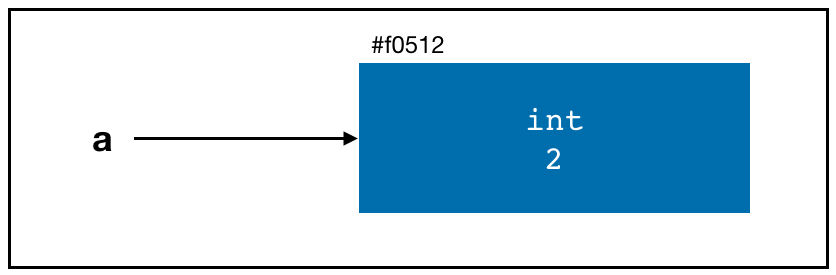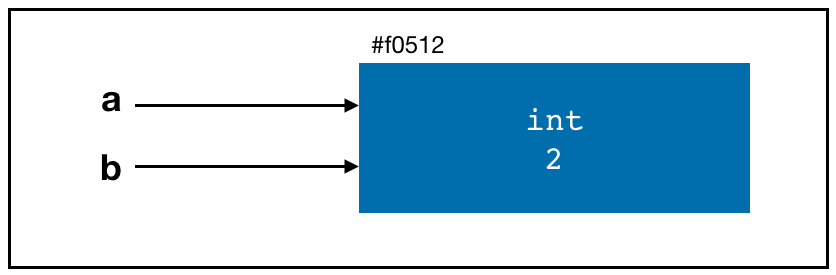## 1. What Is a Variable?

• What is a variable?
• Variable in programming is not the same as variable in a dataset.
• In programming, a variable is a name or a pointer to the object stored in the memory.
a = 2
• In the code above, 2 is an object of type integer (more on that later). It is stored in the computer memory at a certain address (#f0512 in this case).• Whenever we need to access this object (integer 2) in our program, we call a.
a
## 2

## 2. Variable Assignment

• We assign an object to a variable by using =.
• Later on, we will learn about logical operator. In Python (and many other programming languages), the equality operator is ==, not =.
a = 2
b = ab
## 2
a == b
## True

## 3. Binary Operators & Comparisons

Operation Description
a + b Add a and b
a - b Substract b from a
a * b Multiply a by b
a / b Divide a by b
a // b Floor-divide a by b, dropping any fractional remainder
a ** b Raise a to the b power
a & b True if both a and b are True; for integers, take the bitwise AND
a | b True if either a or b is True; for integers, take the bitwise OR
a ^ b True if a is True or b is True, BUT NOT BOTH; for integers, take the bitwise EXCLUSIVE-OR
a == b True if a equals b
a != b True if a is not equal to b
a <= b, a < b True if a is less than (less than or equal) to b
a >= b, a > b True if a is greater than (greater than or equal) to b
a is b True if a and b reference the same Python object
a is not b True if a and b reference different Python objects
• Comparing with is is NOT the same as the == operator:
a = [1, 2, 3]
b = a
c = list(a)
a is b
## True
a is c
## False
a == c
## True

### Exercise

What are the results of the following commands?

a = True
b = False
a & b
a | b
a ^ b
a == b
a != b

Open your Jupyter Notebook to test them out!